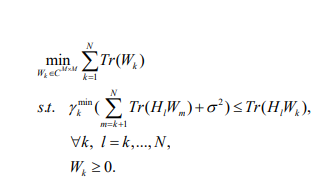# CVX answer in difference from article

Hello;
I’m new in CVX.
I wrote a code of a convex problem and I get answer.
but my objected function respect to answer is way difference from article.
this is my code:

``````M = 5;
UE = 2;
d = randi([1 50],1,UE);
beta = 3.8;
R_min = [1, 1.5, 2, 2.5, 3];
gamga_min=2.^R_min-1;
N0 = 0.01;
power = zeros(length(R_min),1);
h = (sqrt(1/2)*complex(randn(M,UE),randn(M,UE))).*sqrt(d.^(-beta));
[hs, hi] = sort(vecnorm(h));
h = h(:,hi);
for i=1:length(R_min)
cvx_clear
cvx_begin
variable W(M,M,UE) hermitian semidefinite
trace_W = 0;
for ii=1:UE
trace_W = trace_W + trace(W(:,:,ii));
end
minimize(trace_W)
subject to
for k=1:UE
s = 0;
for l=k:UE
H = h(:,l)*h(:,l)';
for m=k+1:UE
s = s + trace(H*W(:,:,m));
end
real(trace(H*W(:,:,k)))>=gamga_min(i)*(real(s)+N0); %#ok<VUNUS>
end
end
cvx_end
power(i) = trace_W;
end
disp(power)
plot(R_min, power)
``````

%%%%%%%%%%%%%%%%%%%%%%%%%%%%%%%%%%%
but power output is in range of 10e4 for different R_min and in article the answer is in range of 10
this is optimization problem in artilce:2 Likes

There is variation in result depending on random numbers. Running it 10 times with different random number draws, here are the low and the high resulting power values:

Low:
10.518550073421324
19.618560360573895
33.730709087357205
57.006863758977957
97.909538362361900

High:
1.0e+05 *
0.143430836355955
0.308233930137237
0.625969817029513
1.264125990435457
2.549542044221298

The high is more than 3 orders of magnitude greater than the low. So aside from any differences in the program and whether it was implemented correctly by you and/or the article authors, obviously the input data values matter in your program…

1 Like